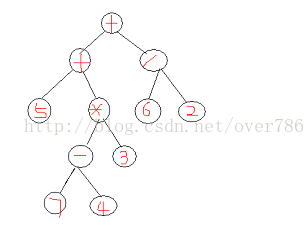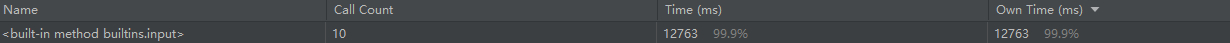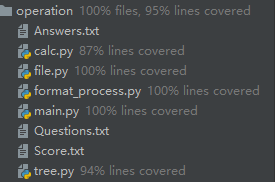# 四则运算

------------恢复内容开始------------

PSP表格

PSP2.1 Personal Software Process Stages 预估耗时（分钟） 实际耗时（分钟）
Planning 计划 40 30
Estimate 估计这个任务需要多少时间 10 10
Development 开发 300 300
Analysis 需求分析 (包括学习新技术) 120 180
Design Spec 生成设计文档 20 20
Design Review 设计复审 20 30
Coding Standard 代码规范 (为目前的开发制定合适的规范) 10 10
Design 具体设计 40 3
Coding 具体编码 240 240
Code Review 代码复审 60 30
Test 测试（自我测试，修改代码，提交修改） 60 50
Reporting 报告 90 120
Test Repor 测试报告 20 20
Size Measurement 计算工作量 20 10
Postmortem & Process Improvement Plan 事后总结, 并提出过程改进计划 60 70

## 用后缀表达式进行运算





栈 :6 5 5


栈 :6 5 5 8


栈 :6 5 40


栈 :6 45


栈 :6 45 3


栈 :6 48


栈 :288


1. lass Calculation:
2.     def eval(op, a, b):                     # 当只有一个操作符的计算
4.         if op == "+":
6.         elif op == "-":
7.             if operator.lt(a, b):           # a是否小于b
8.                 raise NegativeError()       # 当被减数大于减数的时候，抛出异常
9.             else:
11.         elif op == "*":
13.         elif op == "/":
15.             if isinstance(answer, float):    # 当答案为浮点数，转换为分数

20.         num_list = list()
21.         for formula in formula_list:
22.             if isinstance(formula, int) or isinstance(formula, Fraction):
23.                 num_list.append(formula)
24.             else:
25.                 b = num_list.pop()
26.                 a = num_list.pop()
27.                 res = Calculation.eval(formula, a, b)
28.                 num_list.append(res)
29.         return num_list.pop()


## 从中序表达式 转换为 后序表达式

a） 是数字， 直接输出
b） 是运算符
i : “(” 直接入栈
ii : “)” 将符号栈中的元素依次出栈并输出, 直到 “(“, “(“只出栈, 不输出
iii: 其他符号, 将符号栈中的元素依次出栈并输出, 直到 遇到比当前符号优先级更低的符号或者”(“。 将当前符号入栈。

表达式 : 3


表达式 : 3



表达式 : 3



表达式 : 3 2



表达式 : 3 2



表达式 : 3 2 5



表达式 : 3 2 5 -



表达式 : 3 2 5 -



表达式 : 3 2 5 - 6



表达式 : 3 2 5 - 6 *



表达式 : 3 2 5 - 6 * 3



表达式 : 3 2 5 - 6 * 3 / +


## 算术表达式使用递归算法生成二叉树1. #include <iostream>
2. #define MAXSIZE 1000
3.
4. using namespace std;
5.
6. //二叉树结点结构体定义
7. typedef struct BtreeNode{
8.      char data;
9.      struct BtreeNode *lchild;
10. 	 struct BtreeNode *rchild;
11. }BtreeNode;
12.
13. //算术表达式转化二叉树
14. /*
15.    afa为指向表达式字符串的指针
16.    s为要转化的表达式字符串的起始位置
17.    e为要转化的表达式字符串的结束位置的后一个
18. */
19. BtreeNode* afaToBtree(char *afa,int s,int e){
20. 	//如果只有一个数那就是叶子结点了
21. 	if(e-s==1)
22. 	{
23. 		 BtreeNode* bn=(struct BtreeNode*)malloc(sizeof(struct BtreeNode));
24. 		 bn->data=afa[s];
25. 		 bn->lchild=NULL;
26. 		 bn->rchild=NULL;
27. 		 return bn;
28. 	}
29. 	/*
30. 	   local_r记录当前要转化的表达式生成二叉树的根节点操作符的位置
31. 	   flag记录是否当前搜索在括号里面
32.        m_m_p记录当前表达式中括号外面最右边的+、-位置
33. 	   a_s_p记录当前表达式中括号外面最右边的*、/位置
34. 	*/
35.      int local_r=0,flag=0;
36. 	 int m_m_p=0,a_s_p=0;
37. 	 for(int i=s;i<e;i++)
38. 	 {
39. 	    if(afa[i]=='(')flag++;
40. 		else if(afa[i]==')')flag--;
41. 		if(flag==0){
42. 			if(afa[i]=='*'||afa[i]=='/')
43. 				m_m_p=i;
44. 			else if(afa[i]=='+'||afa[i]=='-')
45.                 a_s_p=i;
46. 		}
47. 	 }
48. 	 if((m_m_p==0)&&(a_s_p==0))
49. 		 //如果式子整个有括号如(5-2*3+7)，即括号外面没有操作符，则去掉括号找二叉树
50.          afaToBtree(afa,s+1,e-1);
51. 	 else
52. 	 {
53. 		//如果有+或者-，则根节点为最右边的+或-，否则是最右边的*或/
54.         if(a_s_p>0)local_r=a_s_p;
55. 		else if(m_m_p>0)local_r=m_m_p;
56. 		//确定根节点和根节点的左孩子和右孩子
57. 		BtreeNode* b=(struct BtreeNode*)malloc(sizeof(struct BtreeNode));;
58. 		b->data=afa[local_r];
59.         b->lchild=afaToBtree(afa,s,local_r);
60. 		b->rchild=afaToBtree(afa,local_r+1,e);
61. 	    return b;
62. 	 }
63. }
64.
65. void main(){
66.
67. 	char input[MAXSIZE];
68. 	int len=0;
69. 	//初始化
70. 	memset(input,'\0',sizeof(input));
71.
72. 	cin >> input ;
73.
74.     BtreeNode* myBtree=(struct BtreeNode*)malloc(sizeof(struct BtreeNode));
75.
76. 	//myBtree就是input算术表达式产生的二叉树的根节点(指针类型哦)
77.
78. 	myBtree=afaToBtree(input,0,strlen(input));
79.
80. }


## 运行时间## 异常处理

class NegativeError(Exception):
def __init__(self):
super(NegativeError, self).__init__()


## 代码优化

1. if answer in self.answer:          # 重复度查询，只要计算答案一致，都不加入我们的题库，从结果解决问题
2.       continue


## 代码覆盖率posted @ 2021-10-10 13:19  JATAO丶  阅读(57)  评论(0编辑  收藏  举报